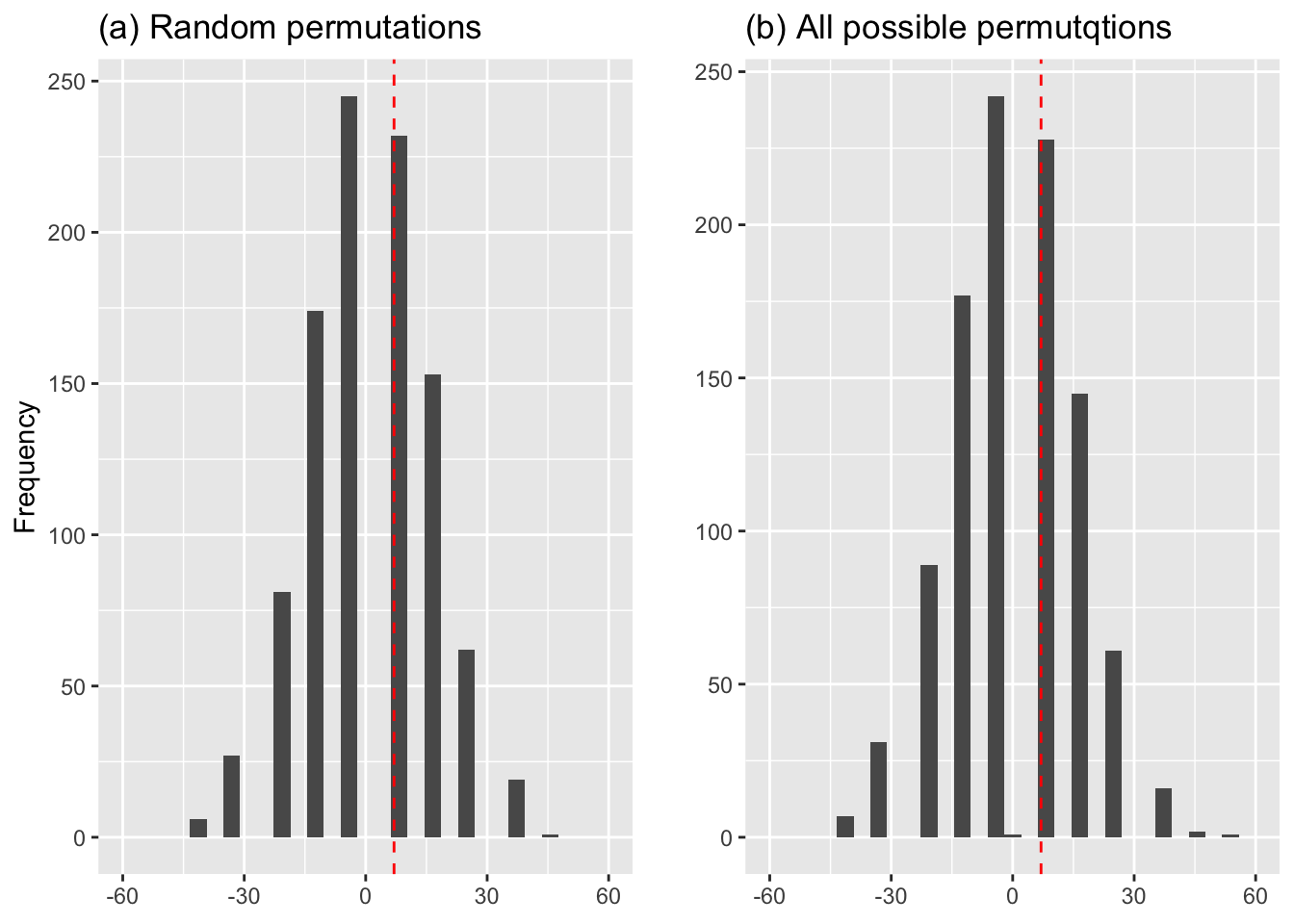Data is contained in 10-2-arms-data-x.csv and was collected at AIMS (African Institute for Mathematical Sciences).

library(ggplot2)
library(ggpubr)
summary(class.frame) # to check variable names, missing data etc
##  gender armcross
##  F:14   L:22
##  M:40   R:32
# Note that text variables are read in as 'factors': categorical variables taking values from a list
attach(class.frame)  # so can use names directly
table(gender,armcross)
##       armcross
## gender  L  R
##      F  5  9
##      M 17 23
# first do hypergeometric, plotted against difference in proportions
# females = white balls, males = black balls, right-arm=balls drawn, x= bottom-left cell

x = seq(14:0)
y = dhyper(x,14,40,32)
female.prop = 100*x/14
male.prop = 100*(32-x)/40
diff=female.prop-male.prop

#change this into 1000 datapoints taking on values of diff
hyper.count= round(1000*y)
cum.hyper.count=cumsum(hyper.count)
diff.hyper.count=rep(0,1000)
for(i in 3:(length(diff)-1)){  # start at first non-zero element
diff.hyper.count[cum.hyper.count[i-1]:cum.hyper.count[i]]  = diff[i]
}
diff.hyper.count.frame=as.data.frame(diff.hyper.count)

# now have to do 1,000 samples without replacement
# trick: vector of arms, then permute
# count number of right-handers in first 14!  YES easy
right.arm = c(rep(0,22),rep(1,32))
diff.sample = rep(0,1000)
for(i in 1:1000){
r=sample(right.arm,54,replace=F)
female.right = sum(r[1:14])
male.right = 32 - female.right
diff.sample[i] = 100*(female.right/14 - male.right/40)
}
diff.sample.frame=as.data.frame(diff.sample)

p <- ggplot(diff.sample.frame) # assign dataframe to plot object, p
p <- p + geom_histogram(aes(diff.sample)) # give it histogram type
p =  p + expand_limits(x=c(-60, 60))
p <- p + labs(x=NULL, y="Frequency", title="(a) Random permutations")
p <- p + geom_vline(xintercept=7, col="red", linetype="dashed") # adds horizontal reference line at x=7

q <- ggplot(diff.hyper.count.frame) # assign dataframe to plot object, q
q <- q + geom_histogram(aes(diff.hyper.count)) # give it histogram type
q =  q + expand_limits(x=c(-60, 60))
q <- q + labs(x=NULL, y="",title="(b) All possible permutqtions")
q <- q + geom_vline(xintercept=7, col="red", linetype="dashed") # adds horizontal reference line at x=7

p <- ggarrange(p,q, ncol=2, nrow=1) # arrange the to plots with ggarrange
## stat_bin() using bins = 30. Pick better value with binwidth.
## stat_bin() using bins = 30. Pick better value with binwidth.
pFigure 10.2 Empirical distribution of difference in proportions of women and men who crossed their arms with their right arm on top: (a) from 1,000 random permutations of arm-crossing, (b) from all possible equally likely permutations of the arm-crossing response. The observed difference in proportions (7%) is indicated by a vertical dashed line.

Hypothesis tests

x=matrix(c(5,17,9,23),2,2)
chisq.test(x)
##
##  Pearson's Chi-squared test with Yates' continuity correction
##
## data:  x
## X-squared = 0.016574, df = 1, p-value = 0.8976
prop.test(c(9,23),c(14,40))
##
##  2-sample test for equality of proportions with continuity
##  correction
##
## data:  c(9, 23) out of c(14, 40)
## X-squared = 0.016574, df = 1, p-value = 0.8976
## alternative hypothesis: two.sided
## 95 percent confidence interval:
##  -0.2744092  0.4101235
## sample estimates:
##    prop 1    prop 2
## 0.6428571 0.5750000
fisher.test(x)
##
##  Fisher's Exact Test for Count Data
##
## data:  x
## p-value = 0.7582
## alternative hypothesis: true odds ratio is not equal to 1
## 95 percent confidence interval:
##  0.1669855 3.0781098
## sample estimates:
## odds ratio
##  0.7555721
x
##      [,1] [,2]
## [1,]    5    9
## [2,]   17   23
# chance of at least 7% diff = chance that female rights is at least  9
1-phyper(8,14,40,32)
##  0.4528027